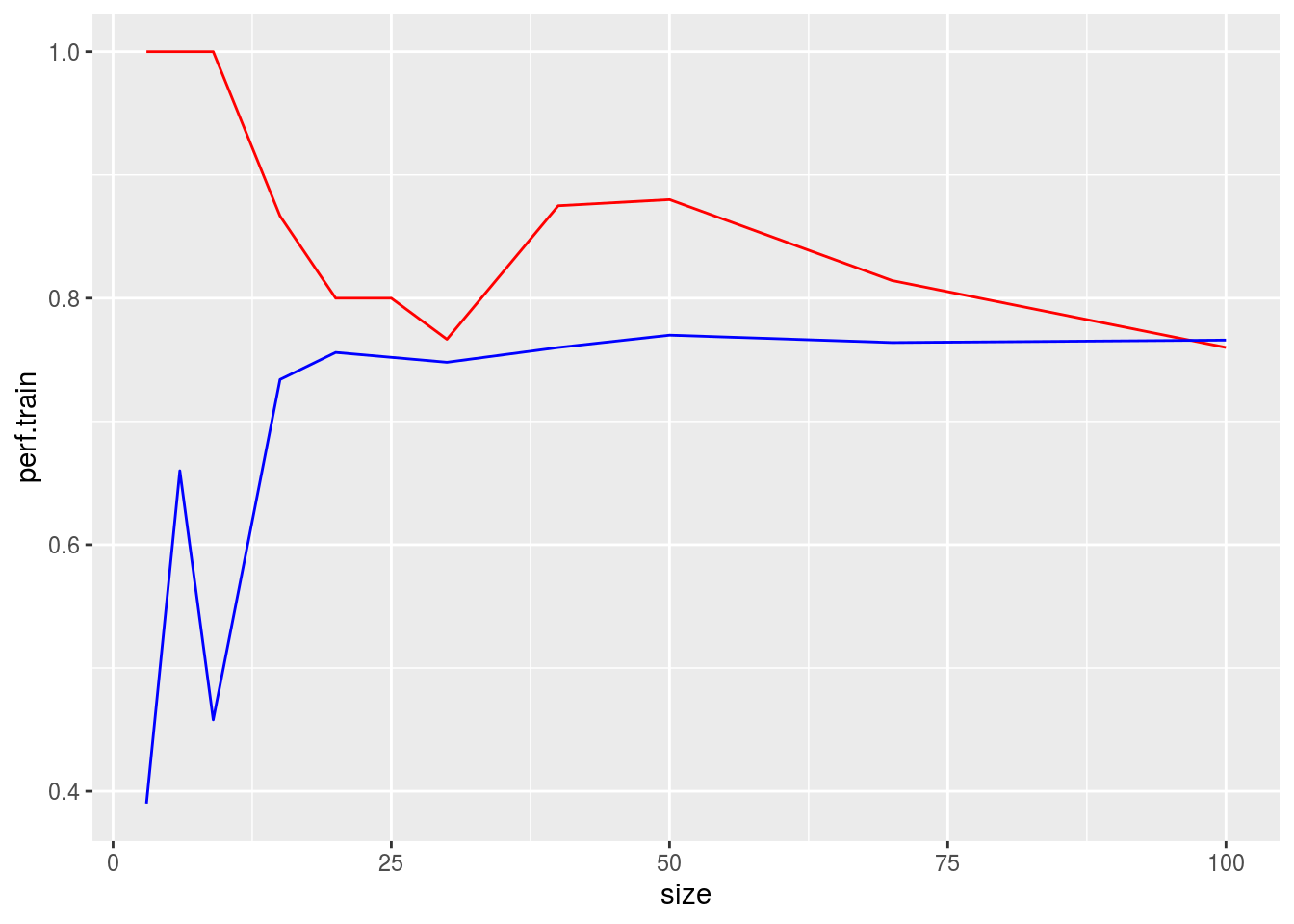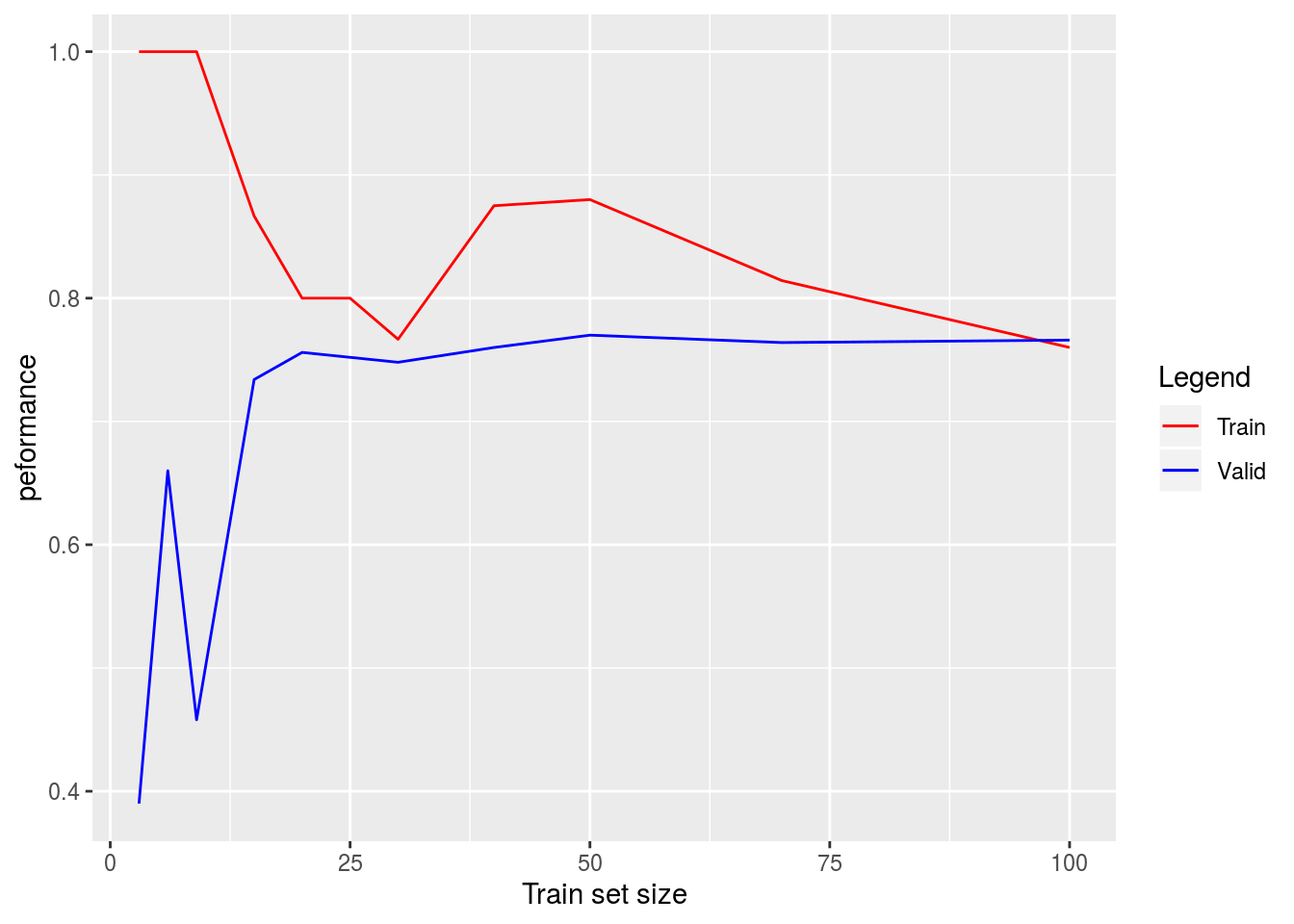### Small training sets and overfitting

titanic <- read.csv("http://guerzhoy.princeton.edu/201s20/titanic.csv")
library(tidyverse)

## Problems 1

### Learning goals

• Practice using R markdown
• Understand that overfitting is good performance on the training set at the expense of good performance on new data
• Understand that overfitting occurs more easily when the data set size is smaller
• Programmatically split data frames
• Practice simulating sampling datasets

We’ll create one training set of size 100, and a validation set of size 500. We’ll only use subsets of the training set throughout. Note that usually, the training set should be as large as possible. Here, we are using a smaller training set for illustration purposes.

set.seed(0)
idx <- sample(1:nrow(titanic))
train.idx <- idx[1:100]
valid.idx <- idx[101:600]

Some functions for computing the performance:

GetTrainValidPerformance <- function(titanic.train, titanic.valid){
fit <- glm(Survived ~ Age + Sex + Pclass, family=binomial, data = titanic.train)
pred.train <- predict(fit, newdata = titanic.train, type = "response") > 0.5
pred.valid <- predict(fit, newdata = titanic.valid, type = "response") > 0.5
c(mean(pred.train == titanic.train$Survived), mean(pred.valid == titanic.valid$Survived))
}

GetTrainValidPerformanceTrSize <- function(train.size, titanic, train.idx, valid.idx){
titanic.valid <- titanic[valid.idx, ]
titanic.train <- titanic[train.idx[1:train.size], ]
GetTrainValidPerformance(titanic.train, titanic.valid)
}

sizes <- c(3, 6, 9, 15, 20, 25, 30, 40, 50, 70, 100)
perf <- sapply(sizes, FUN = GetTrainValidPerformanceTrSize, titanic, train.idx, valid.idx)

Now, let’s add two layers to display the two curves:

perf.data <- data.frame(size = sizes, perf.train = perf[1, ], perf.valid = perf[2, ])
ggplot(data = perf.data, mapping = aes(x = size)) +
geom_line(mapping = aes(y = perf.train), color = "red") +
geom_line(mapping = aes(y = perf.valid), color = "blue")Let’s now add legends, using this technique:

colors <- c("Train" = "red", "Valid" = "blue")
ggplot(data = perf.data, mapping = aes(x = size)) +
geom_line(mapping = aes(y = perf.train, color = "Train")) +
geom_line(mapping = aes(y = perf.valid, color = "Valid"))  +
labs(x = "Train set size", y = "peformance", color = "Legend") +
scale_color_manual(values = colors)